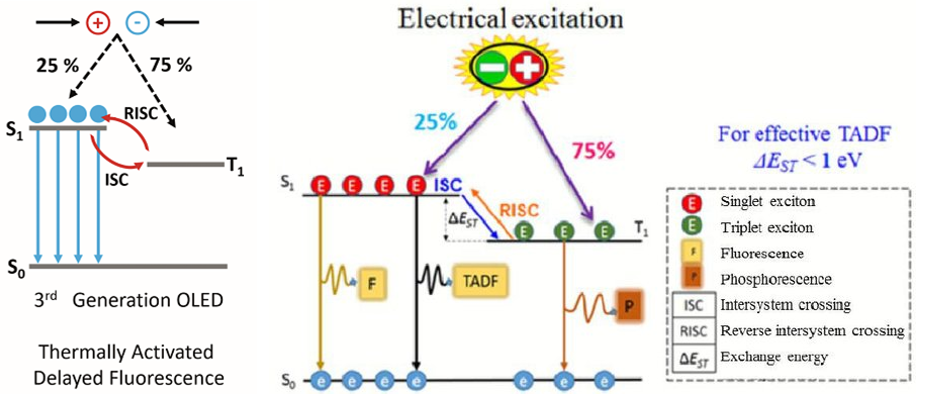## 有机发光

### Singlet State vs Triplet State

Understanding the difference between fluorescence and phosphorescence requires the knowledge of electron spin and the differences between singlet and triplet states. The Pauli Exclusion principle states that two electrons in an atom cannot have the same four quantum numbers $$(n, l, m_{l}, m_{s})$$ and only two electrons can occupy each orbital where they must have opposite spin states. These opposite spin states are called spin pairing. Because of this spin pairing, most molecules do not exhibit a magnetic field and are diamagnetic(抗磁的). In diamagnetic molecules, electrons are not attracted or repelled by the static electric field. Free radicals are paramagnetic(顺磁的) because they contain unpaired electrons have magnetic moments that are attracted to the magnetic field.

Singlet state is defined when all the electron spins are paired in the molecular electronic state and the electronic energy levels do not split when the molecule is exposed into a magnetic field. A doublet state occurs when there is an unpaired electron that gives two possible orientations when exposed in a magnetic field and imparts different energy to the system. A singlet or a triplet can form when one electron is excited to a higher energy level. In an excited singlet state, the electron is promoted in the same spin orientation as it was in the ground state (paired). In a triplet excited stated, the electron that is promoted has the same spin orientation (parallel) to the other unpaired electron.

Singlet, doublet and triplet is derived using the equation for multiplicity $$2S+1$$, where $$S$$ is the total spin angular momentum (sum of all the electron spins).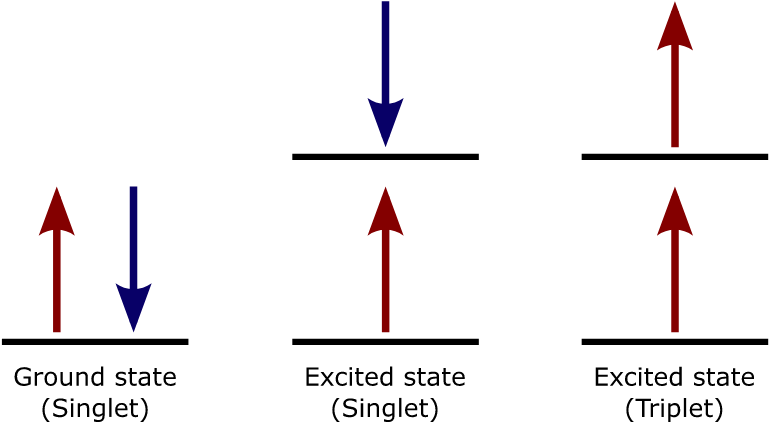### Jablonski Diagrams

The Jablonski diagram that drawn below is a partial energy diagram that represents the energy of photoluminescent molecule in its different energy states. The lowest and darkest horizontal line represents the ground-state electronic energy of the molecule which is the singlet state labeled as $$S_0$$. At room temperature, majority of the molecules in a solution are in this state.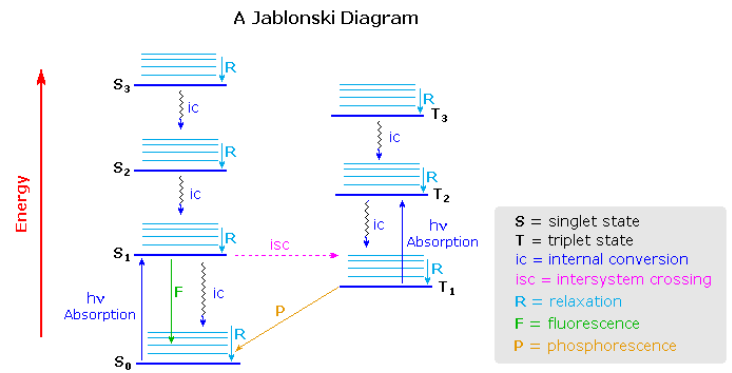The upper darkest line represents the ground vibrational state of the three excited electronic state. The energy of the triplet state is lower than the energy of the corresponding singlet state. (和洪德最大多重度规则有关吗?)There are numerous vibrational levels that can be associated with each electronic state as denoted by the thinner lines. Singlet到Triplet的transition leads to a change in multiplicity and thus has a low probability of occurring which is a forbidden transition. Molecules also go through vibration relaxation to lose any excess vibrational energy that remains when excited to the electronic states ( $$S_1$$ and $$S_2$$ ) as demonstrated in wavy lines. The knowledge of forbidden transition is used to explain and compare the peaks of absorption and emission.

### Relaxation and Fluorescence

When an electron is promoted to an electronic excited state, it often ends up in an excited vibrational state as well. Thus, some of the energy put into electronic excitation is immediately passed into vibrational energy. Vibrational energy, however, doesn't just travel in photons. It can be gained or lost through molecular collisions and heat transfer.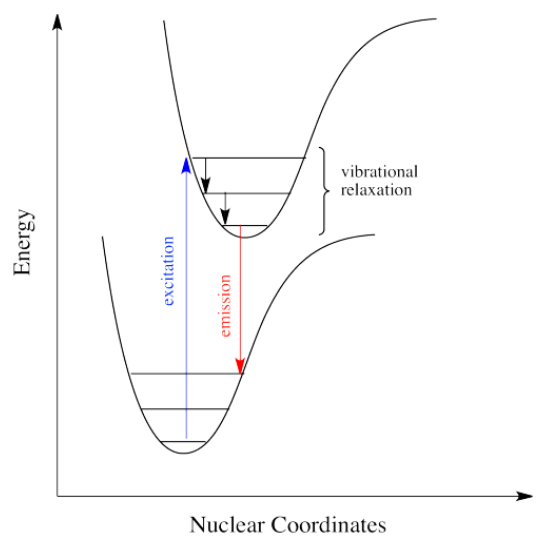Just how does a molecule undergo vibrational relaxation? Vibrational energy is the energy used to lengthen or shorten bonds, or to widen or squeeze bond angles.
(1) 大分子：Given a big enough molecule, some of this vibrational energy could be transferred into bond lengths and angles further away from the electronic transition.
(2) 小分子：Otherwise, if the molecule is small, it may transfer some of its energy in collisions with other molecules.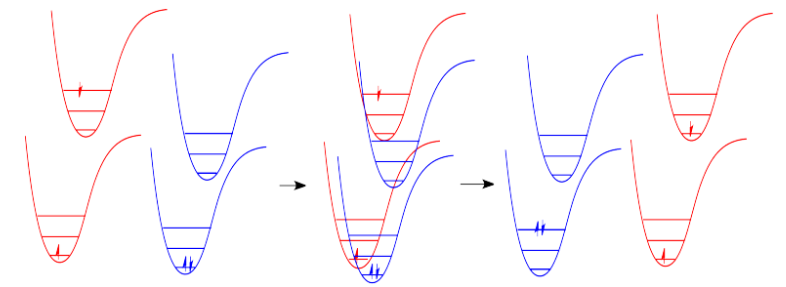In molecules, as one molecule drops to a lower vibrational state, the other will hop up to a higher vibrational state with the energy it gains. In the drawing below, the red molecule is in an electronic excited and vibrational state. In a collision, it transfers some of its vibrational energy to the blue molecule.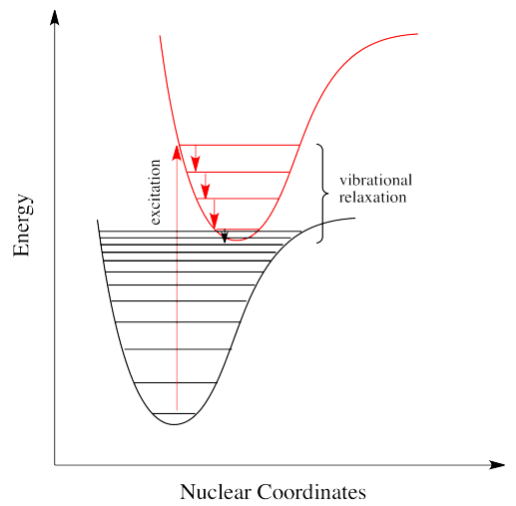If electrons can get to a lower energy state, and give off a little energy at a time, by hopping down to lower and lower vibrational levels, do they need to give off a giant photon at all? Maybe they can relax all the way down to the ground state via vibrational relaxation. That is certainly the case. Given lots of vibrational energy levels, and an excited state that is low enough in energy so that some of its lower vibrational levels overlap with some of the higher vibrational levels of the ground state, the electron can hop over from one state to the other, without releasing a photon.

This event is called a "radiationless transition", because it occurs without release of a photon. The electron simply slides over from a low vibrational state of the excited electronic state to a high vibrational state of the electronic ground state. If the electron simply keeps dropping a vibrational level at a time back to the ground state, the process is called "internal conversion".

Internal conversion has an important consequence. Because the absorption of UV and visible light can result in energy transfer into vibrational states, much of the energy that is absorbed from these sources is converted into heat. That can be a good thing if you happen to be a marine iguana trying to warm up in the sun after a plunge in the icy Pacific. It can also be a tricky thing if you are a process chemist trying to scale up a photochemical reaction for commercial production of a pharmaceutical, because you have to make sure the system has adequate cooling available.

There is a very similar event, called intersystem crossing, that leads to the electron getting caught between the excited state and the ground state. Just as, little by little, vibrational relaxation can lead the electron back onto the ground state energy surface, it can also lead the electron into states that are intermediate in energy.

For example, suppose an organic molecule undergoes electronic excitation. Generally, organic molecules have no unpaired electrons. Their ground states are singlet states. According to one of our selection rules for electronic excitation, the excited state must also have no unpaired electrons. In other words, the spin on the electron that gets excited is the same after excitation as it was before excitation.

However, that's not the lowest possible energy state for that electron. When we think about atomic orbital filling, there is a rule that governs the spin on the electrons in degenerate orbitals: in the lowest energy state, spin is maximized (Hund's rule). In other words, when we draw a picture of the valence electron configuration of nitrogen, we show nitrogen's three p electrons each in its own orbital, with their spins parallel.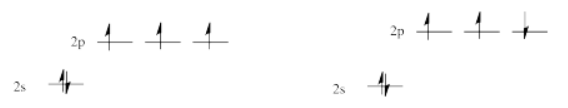The picture with three unpaired electrons, all with parallel spins, shows a nitrogen in the quartet spin state. Having one of those spins point the other way would result in a different spin state. One pair of electrons in the $$p$$ level would be spin-paired, one up and one down, even though they are in different $$p$$ orbitals. That would leave one electron without an opposite partner. The nitrogen would be in a doublet spin state. That is not what happens. The spin state on the left is lower in energy than the state on the right. That's just one of the rules of quantum mechanics (Hund's rule): maximize spin when orbitals are singly occupied.

It's the same in a molecule with the triplet state lower in energy than the singlet state. Why didn't the electron get excited to the triplet state in the first place? That's against the rules. But sliding down vibrationally onto the triplet state from the singlet excited state is not, because it doesn't involve absorption of a photon.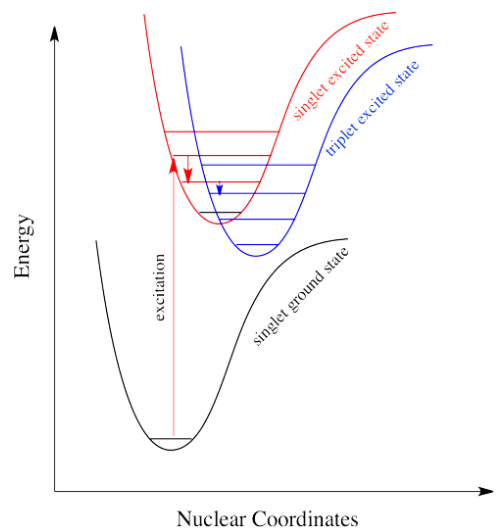Intersystem crossing can have important consequences in reaction chemistry because it allows access to triplet states that are not normally avaiable in many molecules. Because triplet states feature unpaired electrons, their reactivity is often typified by radical processes. That means an added suite of reactions can be accessed via this process.

Intersystem crossing is one way a system can end up in a triplet excited state. Even though this state is lower in energy than a singlet excited state, it cannot be accessed directly via electronic excitation because that would violate the spin selection rule ($$\Delta S=0$$). That's where the electron gets stuck, though. The quick way back down to the bottom is by emitting a photon, but because that would involve a change in spin state, it is not allowed.(可通过spin-orbit coupling进行) Realistically speaking, that means it takes a long time. By "a long time", we might mean a few seconds, several minutes, or possibly even hours. Eventually, the electron can drop back down, accompanied by the emission of a photon. This situation is called "phosphorescence".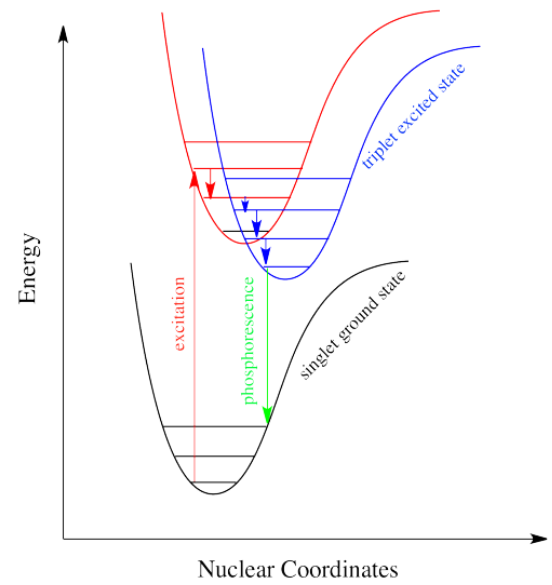Molecules that display phosphorescence are often incorporated into toys and shirts so that they will glow in the dark.

### Molecular Absorption of Light

When we were talking about the various sorts of molecular orbitals present in organic compounds earlier, you will have come across this diagram showing their relative energies: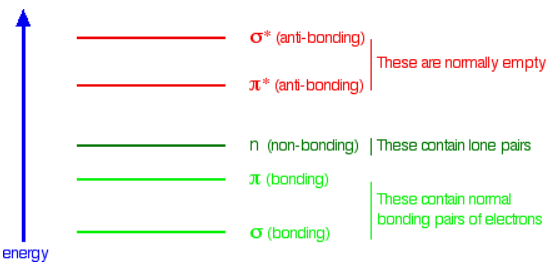When light passes through the compound, energy from the light is used to promote an electron from a bonding or non-bonding orbital into one of the empty anti-bonding orbitals. The possible electron jumps that light might cause are: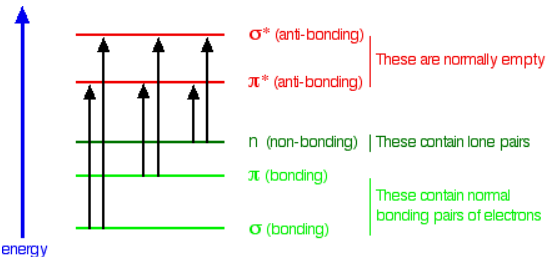In each possible case, an electron is excited from a full orbital into an empty anti-bonding orbital. Each jump takes energy from the light, and a big jump obviously needs more energy than a small one. Each wavelength of light has a particular energy associated with it. If that particular amount of energy is just right for making one of these energy jumps, then that wavelength will be absorbed - its energy will have been used in promoting an electron.

An absorption spectrometer works in a range from about 200 nm (in the near ultra-violet) to about 800 nm (in the very near infra-red). Only a limited number of the possible electron jumps absorb light in that region. Look again at the possible jumps. This time, the important jumps are shown in black, and a less important one in grey. The grey dotted arrows show jumps which absorb light outside the region of the spectrum we are working in.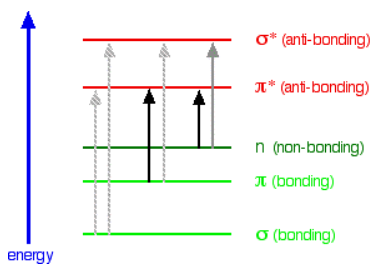Remember that bigger jumps need more energy and so absorb light with a shorter wavelength. The jumps shown with grey dotted arrows absorb UV light of wavelength less that 200 nm. The important jumps are:

• from $$\pi$$bonding orbitals to $$\pi$$anti-bonding orbitals;
• from non-bonding orbitals to $$\pi$$ anti-bonding orbitals;
• from non-bonding orbitals to sigma anti-bonding orbitals.

That means that in order to absorb light in the region from 200 - 800 nm (which is where the spectra are measured), the molecule must contain either $$\pi$$ bonds or atoms with non-bonding orbitals. Remember that a non-bonding orbital is a lone pair on, say, oxygen, nitrogen or a halogen. Groups in a molecule which absorb light are known as chromophores.

The diagram below shows a simple UV-visible absorption spectrum for buta-1,3-diene - a molecule we will talk more about later. Absorbance (on the vertical axis) is just a measure of the amount of light absorbed. The higher the value, the more of a particular wavelength is being absorbed.

### UV-visible Absorption spectrum

he diagram below shows a simple UV-visible absorption spectrum for buta-1,3-diene - a molecule we will talk more about later. Absorbance (on the vertical axis) is just a measure of the amount of light absorbed. The higher the value, the more of a particular wavelength is being absorbed.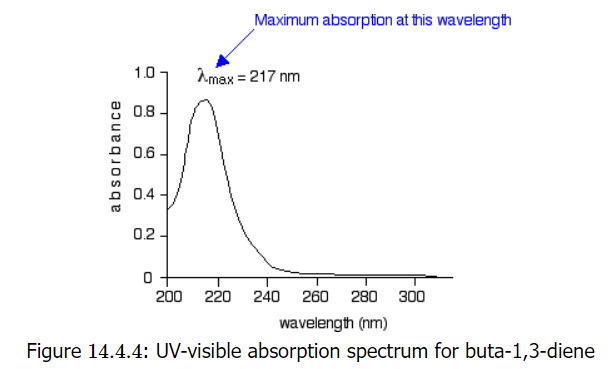You will see that absorption peaks at a value of 217 nm. This is in the ultra-violet and so there would be no visible sign of any light being absorbed - buta-1,3-diene is colorless. In buta-1,3-diene, CH2=CH-CH=CH2, there are no non-bonding electrons. That means that the only electron jumps taking place (within the range that the spectrometer can measure) are from $$\pi$$ bonding to $$\pi$$ anti-bonding orbitals.

A chromophore such as the carbon-oxygen double bond in ethanal, for example, obviously has $$\pi$$ electrons as a part of the double bond, but also has lone pairs on the oxygen atom. That means that both of the important absorptions from the last energy diagram are possible. You can get an electron excited from a $$\pi$$ bonding to a $$\pi$$ anti-bonding orbital, or you can get one excited from an oxygen lone pair (a non-bonding orbital) into a $$\pi$$ anti-bonding orbital.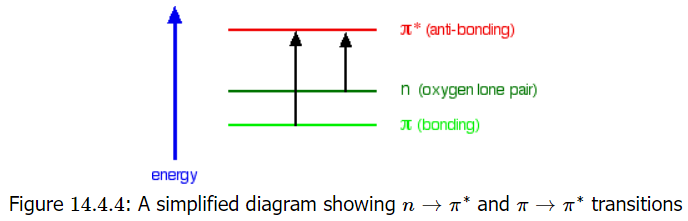The non-bonding orbital has a higher energy than a $$\pi$$ bonding orbital. That means that the jump from an oxygen lone pair into a $$\pi$$ anti-bonding orbital needs less energy. That means it absorbs light of a lower frequency and therefore a higher wavelength. For example, ethanal can therefore absorb light of two different wavelengths:

• the $$\pi$$ bonding to $$\pi$$ anti-bonding absorption peaks at 180 nm. These $$n \rightarrow \pi^{*}$$ transitions involve moving an electron from a nonbonding electron pair to a antibonding $$\pi^{*}$$ orbital. They tend to have molar absorbtivities less than 2000;
• the non-bonding to $$\pi$$ anti-bonding absorption peaks at 290 nm. These $$\pi \rightarrow \pi^{*}$$ transitions involve moving an electron from a bonding $$\pi^{*}$$ orbital to an antibonding $$\pi^{*}$$ orbital. They tend to have molar absorptivities on the order of 10,000.
• 上面好像写反了。

### Conjugation and Delocalization

Consider these three molecules: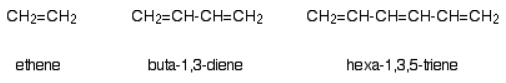Ethene contains a simple isolated carbon-carbon double bond, but the other two have conjugated double bonds. In these cases, there is delocalization of the $$\pi$$ bonding orbitals over the whole molecule. Now look at the wavelengths of the light which each of these molecules absorbs.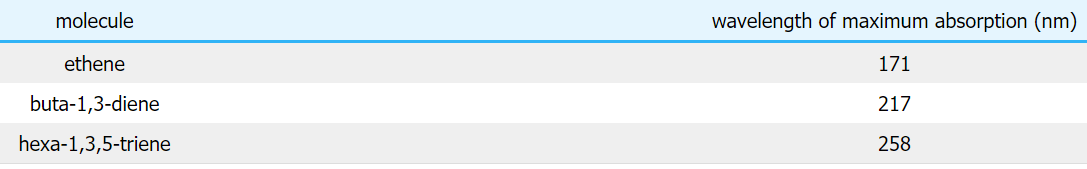All of the molecules give similar UV-visible absorption spectra - the only difference being that the absorptions move to longer and longer wavelengths as the amount of delocalization in the molecule increases. Why is this? You can actually work out what must be happening.

• The maximum absorption is moving to longer wavelengths as the amount of delocalization increases.
• Therefore maximum absorption is moving to shorter frequencies as the amount of delocalization increases.
• Therefore absorption needs less energy as the amount of delocalization increases.
• Therefore there must be less energy gap between the bonding and anti-bonding orbitals as the amount of delocalization increases.

Compare ethene with buta-1,3-diene. In ethene, there is one $$\pi$$ bonding orbital and one $$\pi$$ anti-bonding orbital. In buta-1,3-diene, there are two $$\pi$$ bonding orbitals and two $$\pi$$ anti-bonding orbitals. This is all discussed in detail on the introductory page that you should have read.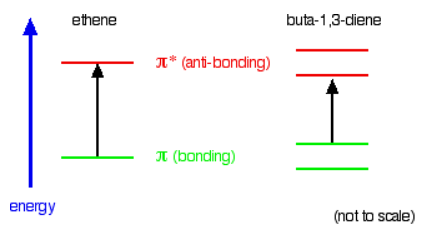The highest occupied molecular orbital is often referred to as the HOMO - in these cases, it is a $$\pi$$ bonding orbital. The lowest unoccupied molecular orbital (the LUMO) is a $$\pi$$ anti-bonding orbital. Notice that the gap between these has fallen. It takes less energy to excite an electron in the buta-1,3-diene case than with ethene. In the hexa-1,3,5-triene case, it is less still.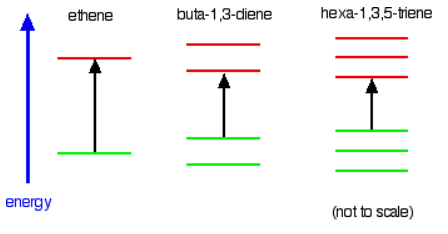If you extend this to compounds with really massive delocalization, the wavelength absorbed will eventually be high enough to be in the visible region of the spectrum, and the compound will then be seen as colored. A good example of this is the orange plant pigment, beta-carotene - present in carrots, for example.

(1) Why is beta-carotene orange?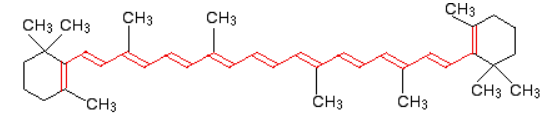Beta-carotene has the sort of delocalization that we've just been looking at, but on a much greater scale with 11 carbon-carbon double bonds conjugated together. The diagram shows the structure of beta-carotene with the alternating double and single bonds shown in red.The more delocalization there is, the smaller the gap between the highest energy $$\pi$$ bonding orbital and the lowest energy $$\pi$$ anti-bonding orbital. To promote an electron therefore takes less energy in beta-carotene than in the cases we've looked at so far - because the gap between the levels is less. Beta-carotene absorbs throughout the ultra-violet region into the violet - but particularly strongly in the visible region between about 400 and 500 nm with a peak about 470 nm.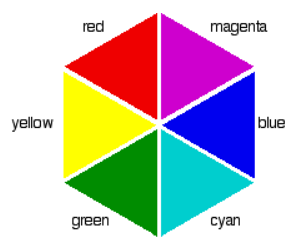Color Wheel
(2)  CB/VB 和LUMO/HOMO对比[Thomas Jüstel-PPT]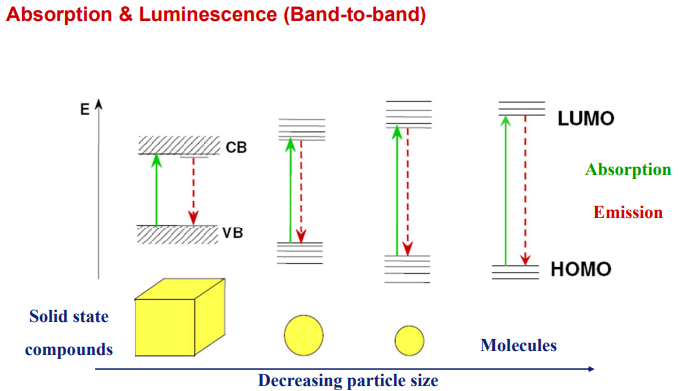### Franck-Condon: Electronic and Vibrational Coupling

So far, we have come across one big rule of photon absorbance. To be absorbed, a photon's energy has to match an energy difference within the compound that is absorbing it. Different wavelengths would be able to promote different electrons, depending on the energy difference between an occupied electronic energy level and an unoccupied one. Other types of electromagnetic radiation would not be able to promote an electron, but they would be coupled to other events. For example, absorption of infrared light is tied to vibrational energy levels. Microwave radiation is tied to rotational energy levels in molecules. Thus, one reason a photon may or may not be absorbed has to do with whether its energy corresponds to the available energy differences within the molecule or ion that it encounters.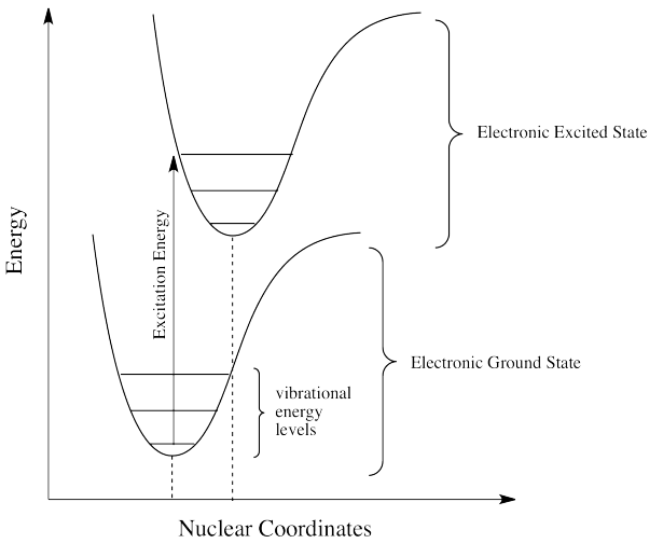Photons face other limitations. One of these is a moderate variation on our main rule. It is called the Frank Condon Principle. According to this idea, when an electron is excited from its normal position, the ground state, to a higher energy level, the optimal positions of atoms in the molecule may need to shift. Because electronic motion is much faster than nuclear motion, however, any shifting of atoms needed to optimize positions as they should be in the excited state will have to wait until after the electron gets excited. In that case, when the electron lands and the atoms aren't yet in their lowest energy positions for the excited state, the molecule will find itself in an excited vibrational state as well as an excited electronic state. That means the required energy for excitation does not just correspond to the difference in electronic energy levels; it is fine-tuned to reach a vibrational energy level, which is quantized as well.

### 影响荧光的主要因素

(1) 荧光助色团与荧光消色团

(2) 增加稠合环可增强荧光

(3) 提高分子的刚性可增强荧光

(4) 激发态电子组态的影响

(5) 重原子将导致荧光量子产率的降低

(6) 溶剂极性的影响

(7) 其它影响因素

## Lanthanide Complexes

### TR-FRET用稀土螯合物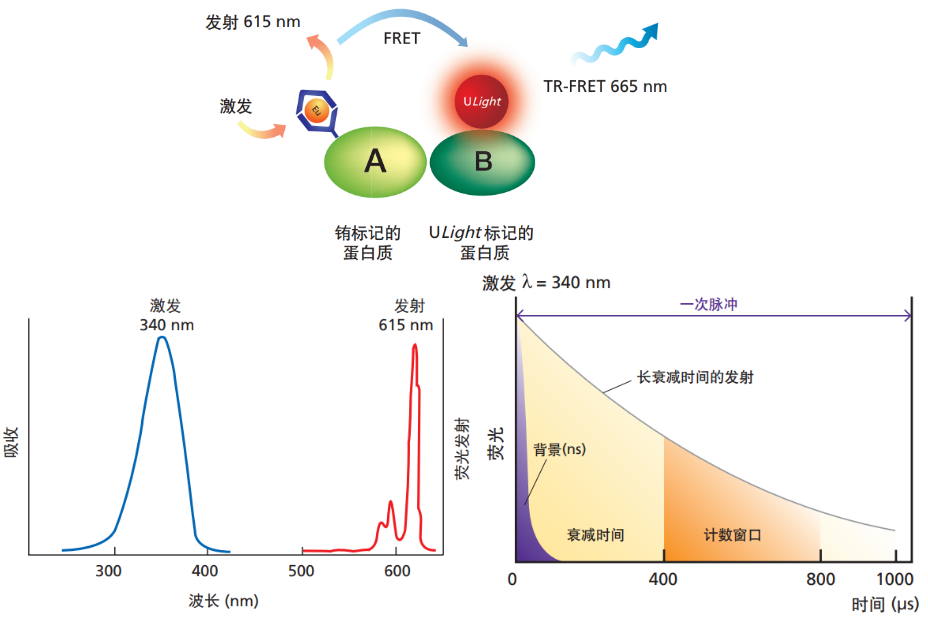(1) 荧光寿命增加了100,000 倍

(2) 较大的斯托克斯位移

(3) 荧光峰的峰形尖锐

1

2

3

## OLED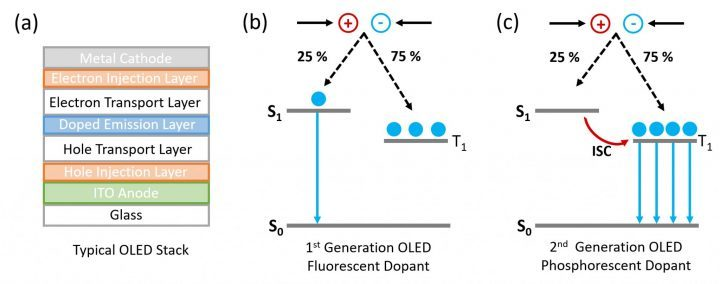Fluorescence: S(单线态)到S的跃迁；
Phosphorescence: T(单线态)到S的跃迁；